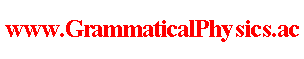since 2006 Help　Sitemap < Forum > < Definition > < Coordinate System > I use the word ‘coordinate system' in a little broader meaning than the ordinary meaning. The coordinate system which I say is a general mapping 　　　　　　　　which maps a mathematical notion to a physical notion. Specifically, as the coordinate system of a physical theory, I think of a mapping 　　　　　　 which maps a mathematical notion to a history of the physical system. For example, a physical unit is a coordinate system in my broad meaning. It is because the unit of length cm 　　　　　　　　　can be thought of 　　　　　　　　　　　　　　as the mapping 　　　　　　　　　　　　　　　　which maps each real number x to a length x centimeters 　　　　　　　　and the unit of time s 　　　　　　　　　　　can be thought of 　　　　　　　　　　　　　　　　as the mapping 　　　　　　　　　　　　　　　　　　which maps each real number t to the time t seconds.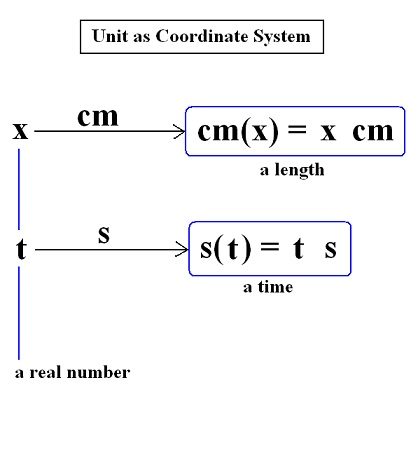By using the units cm and s, I define the Cartesian coordinate system D and the time coordinate system ‘clock' 　as shown in these figures.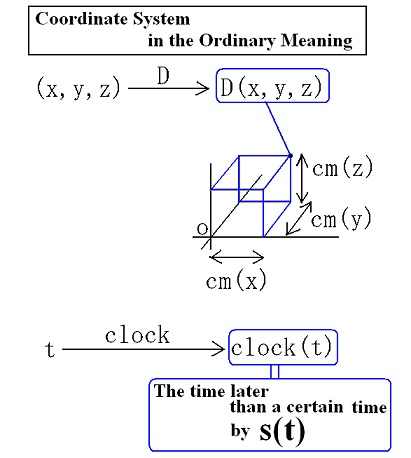D is the mapping 　　　which maps each real row vector (x,y,z) 　　　　　　　to a spatial point whose Cartesian coordinate is (x,y,z), and ‘clock’ is the mapping 　　　　　　　　　which maps each real number t 　　　　　　　　　　　　　to the time later by t seconds than the arbitrarily given original time. Therefore, Both D and clock are coordinate systems in my broad meaning. As the coordinate systems of the physical theories, I can mention the coordinate system of the classical mechanics for a particle 　　　　　　　　　and the coordinate system of the quantum mechanics for it. The coordinate system Mc of the classical mechanics for a particle is defined as the mapping 　　　　　　　　which maps each function χ from R to R3 　 　　　 　　　　　　　 to a history Mc(χ) of the position of the particle where Mc(χ) is the following proposition. “For any real number t, the position of the particle is D(χ(t)) at the time clock(t).”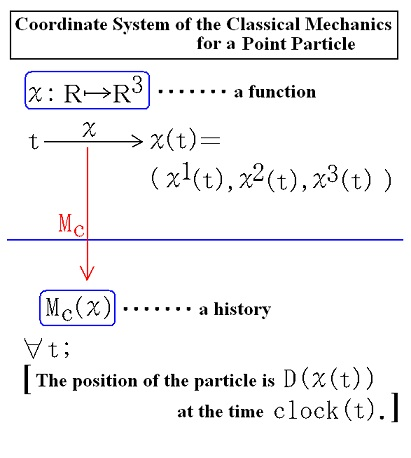The coordinate system Mq of the quantum mechanics for a particle is the mapping 　　which maps each function Ψ from R4 to C 　 　　　　　to a history Mq(Ψ) of the quantum state of the particle where Mq(Ψ) is the following proposition. “For any real number t, 　the quantum state of the particle is state(Ψ(□,t)) at the time clock(t).” Please notice that in this statement 　　　　　　　　　　　　Ψ(□,t) is a mapping from R3 to C 　　　　　　　　　　　　　　　　　and is defined by the equation [Ψ(□,t)](x,y,z)=Ψ(x,y,z,t) 　　　　　　　　　　　　　and ‘state’ is a mapping which maps each function from R3 to C 　　　　　　　　　　　　　　　　　　　　　　　　　　　　　　　　　to a quantum state 　　　　　　　　　　　　　　　　　　　　　and is a coordinate system in my broad meaning.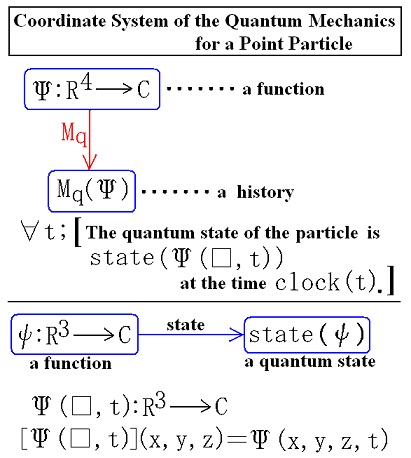--- The content of this article was presented at APS+JPS 2006 Autumn Meeting. Author Yuichi Uda, Write start at 2016/04/03/12:03JST, Last edit at 2016/04/03/14:08JST